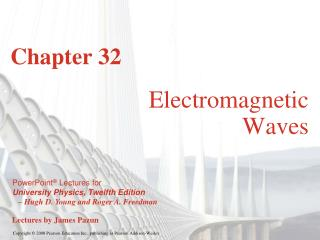Download PresentationChapter 32

# Chapter 32 - PowerPoint PPT PresentationDownload Presentation## Chapter 32

- - - - - - - - - - - - - - - - - - - - - - - - - - - E N D - - - - - - - - - - - - - - - - - - - - - - - - - - -
##### Presentation Transcript

1. Chapter 32 Electromagnetic Waves

2. Goals for Chapter 32 • To do an overview of Maxwell’s equations and electromagnetic waves • To study sinusoidal electromagnetic waves • To consider the passage of electromagnetic waves through matter • To determine the energy and momentum of electromagnetic waves • To observe wave addition, the formation of a standing electromagnetic wave

3. Introduction • If an electric field vector propagates, it generates a magnetic field vector. Or, is it the other way? • The “chicken and the egg” argument of which disturbance causes the other aside, this is often a favorite portion of a first course in physics. Electromagnetic waves, at least in the form of light, are common to many of our daily experiences. Even without vision, you can stand in the sun wearing a dark shirt and perceive electromagnetic waves.

4. Maxwell’s equations • After Ampere and Faraday came James Clark Maxwell. He penned a set of four equations that draw Gauss, Ampere, and Faraday’s laws together in a comprehensive description of the behavior of electromagnetic waves. • The four elegant equations are found at the bottom of page 1093. The photo of Maxwell even seems to be pondering what he had written.

5. Electromagnetic waves are ubiquitous • If you tried to cite all the places you notice electromagnetic waves in your classroom, you would conclude in a few minutes that they are everywhere.

6. Creating electromagnetic waves • Consider Figure 32.3. • A pulse of electricity oscillates in a conductor. Created by the moving electric field, a magnetic field propagates at right angles.

7. Electromagnetic waves occur over a wide range • Where wavelength is large, frequency is small. • The range extends from low energy and frequency (radio and television) to high energy and small wavelength (gamma rays).

8. The visible spectrum • The visible spectrum is a very small range compared to the entire electromagnetic spectrum. • Visible light extends from red light at 700 nm to violet light at 400 nm.

9. The propagation of electromagnetic waves • Consider Figures 32.5 and 32.6. • The wave front moves at speed c, equal to 2.99 × 108 m/s.

10. Propagation of electromagnetic waves II • The entire process can be considered as alternating applications of Faraday’s laws and Ampere’s Law. • Consider Figures 32.7 and 32.8 below.

11. Propagation of electromagnetic waves III • The wave is transverse, moving at unchanging c in a vacuum, with electric and magnetic fields in a definite ratio, and requiring no medium (like water or air). • The right-hand rule applies—see Figure 32.9 below.

12. The wave equation follows from Ampere and Faraday • Because the wave propagates by application of Ampere and Faraday’s Laws (as mentioned earlier), we can combine those relationships and obtain a second-order differential wave equation. • Refer to Figures 32.10 and 32.11 below.

13. Electromagnetic waves may be treated as plane waves • Far enough from the source and considering one polarization of the vector planes only, the representations of electric and magnetic fields may be treated as orthogonal and sinusoidal waves. • Read Problem-Solving Strategy 32.1. • Consider Figures 32.12 and 32.13 below.

14. Intrinsicproperties and extrinsic motion of an EM wave • Follow Example 32.1. • Figure 32.15 illustrates Example 32.1. • Follow Example 32.2.

15. Energy in an EM wave, the Poynting vector • The energy in an electromagnetic wave can be considered by thinking of energy densities. • Consider Figures 32.17 and 32.18 below. • Follow Examples 32.3 and 32.4.

16. “Solar wind” • Although we don’t normally think of photons and momentum in the same moment, there is power and pressure in light. • Consider Figures 32.20 and 32.21 below. • Follow Example 32.5, illustrated by Figure 32.21.

17. Standing EM waves • Thinking of “standing EM waves” might seem just as interesting as photons and momentum, but a microwave oven is using just that principle. An EM wave can be reflected so waves can add constructively and resonate in a cavity. • Consider Figures 32.22 and 32.23 below. • Follow Examples 32.6 and 32.7.